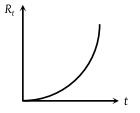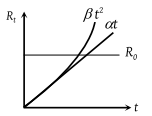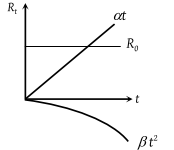The resistance Rt of a conductor varies with temperature t as shown in the figure. If the variation is represented by ${R}_{t}={R}_{0}\left[1+\alpha t+\beta {t}^{2}\right]$, then(1) α and β are both negative

(2) α and β are both positive

(3) α is positive and β is negative

(4) α is negative and β are positive

(3) Since the value of R continuously increases, both α and β must be positive.

Actually the components of the given equation are as followsIt α is positive, β is negative, the component will be shown in the following graph.In this case, the value of R will not increase continuously. Hence the correct option is (3).

Difficulty Level:

• 15%
• 54%
• 26%
• 7%